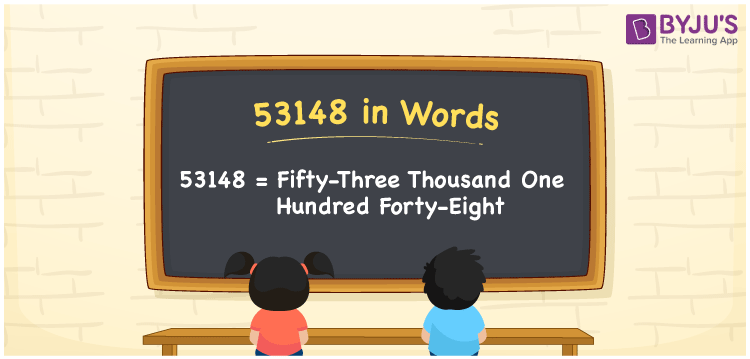# 53148 in Words

The number 53148 in words is “fifty-three thousand one hundred forty-eight”. The place value system plays a major role in writing the number names. For instance, if you earned Rs. 53148 in four months, you can write it as “I earned Rs. Fifty-three thousand one hundred forty-eight in four months”. Also, 53148 is a cardinal number.

 53148 in Words: Fifty-three Thousand One Hundred Forty-eight. Fifty-three Thousand One Hundred Forty-eight in Numerical Form: 53148.

## 53148 in English Words## How to Write 53148 in Words?

The place values of 53148 are presented in the below table.

 Ten-thousands Thousands Hundreds Tens Ones 5 3 1 4 8

The expanded form of 53148 is as follows:

= 5 × Ten thousand + 3 × Thousand + 1 × Hundred + 4 × Ten + 8 × One

= 5 × 10000 + 3 × 1000 + 1 × 100 + 4 × 10 + 8 × 1

= 50000 + 3000 + 100 + 40 + 8

= 53148

= Fifty-three thousand one hundred forty-eight

Hence, 53148 in words is fifty-three thousand one hundred forty-eight.

53148 in words – Fifty-three thousand one hundred forty-eight

Is 53148 an odd number? – No

Is 53148 an even number? – Yes

Is 53148 a perfect square number? – No

Is 53148 a perfect cube number? – No

Is 53148 a prime number? – No

Is 53148 a composite number? – Yes

## Frequently Asked Questions on 53148 in Words

Q1

### Write 53148 in words.

53148 in words is fifty-three thousand one hundred forty-eight.

Q2

### Simplify 53000 + 148, and express it in words.

Simplifying 53000 + 148, we get 53148. Hence, 53148 in words is fifty-three thousand one hundred forty-eight.

Q3

### Is 53148 a prime number?

No, 53148 is not a prime number.